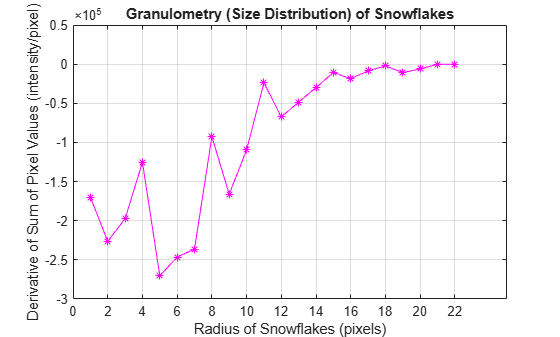Documentation

### This is machine translation

Mouseover text to see original. Click the button below to return to the English version of the page.

To view all translated materials including this page, select Country from the country navigator on the bottom of this page.

## Granulometry of Snowflakes

This example shows how to calculate the size distribution of snowflakes in an image by using granulometry. Granulometry determines the size distribution of objects in an image without explicitly segmenting (detecting) each object first.

Read in the 'snowflakes.png' image, which is a photograph of snowflakes.

```I = imread('snowflakes.png'); imshow(I)```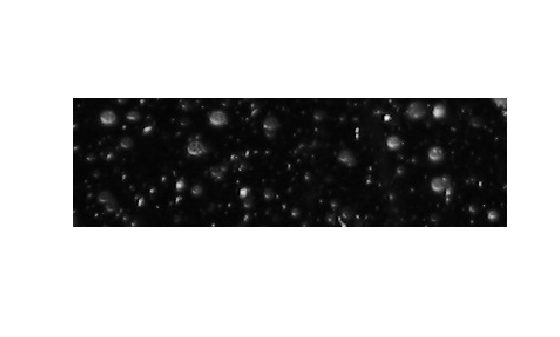### Enhance Contrast

Your first step is to maximize the intensity contrast in the image. You can do this using the `adapthisteq` function, which performs contrast-limited adaptive histogram equalization. Rescale the image intensity using the `imadjust` function so that it fills the data type's entire dynamic range.

```claheI = adapthisteq(I,'NumTiles',[10 10]); claheI = imadjust(claheI); imshow(claheI)```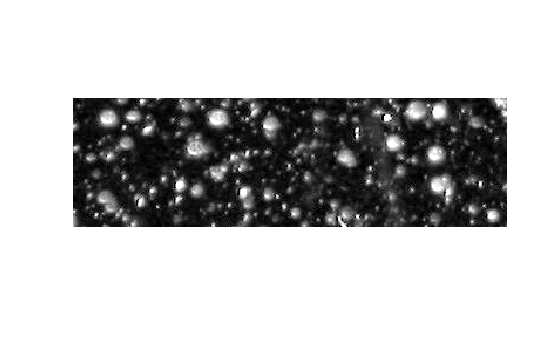### Determine Intensity Surface Area Distribution in Enhanced Image

Granulometry estimates the intensity surface area distribution of snowflakes as a function of size. Granulometry likens image objects to stones whose sizes can be determined by sifting them through screens of increasing size and collecting what remains after each pass. Image objects are sifted by opening the image with a structuring element of increasing size and counting the remaining intensity surface area (summation of pixel values in the image) after each opening.

Choose a counter limit so that the intensity surface area goes to zero as you increase the size of your structuring element. For display purposes, leave the first entry in the surface area array empty.

```radius_range = 0:22; intensity_area = zeros(size(radius_range)); for counter = radius_range remain = imopen(claheI, strel('disk', counter)); intensity_area(counter + 1) = sum(remain(:)); end figure plot(intensity_area, 'm - *') grid on title('Sum of pixel values in opened image versus radius') xlabel('radius of opening (pixels)') ylabel('pixel value sum of opened objects (intensity)')```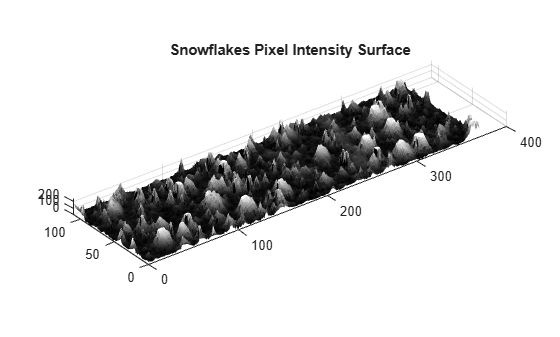### Calculate First Derivative of Distribution

A significant drop in intensity surface area between two consecutive openings indicates that the image contains objects of comparable size to the smaller opening. This is equivalent to the first derivative of the intensity surface area array, which contains the size distribution of the snowflakes in the image. Calculate the first derivative with the `diff` function.

```intensity_area_prime = diff(intensity_area); plot(intensity_area_prime, 'm - *') grid on title('Granulometry (Size Distribution) of Snowflakes') ax = gca; ax.XTick = [0 2 4 6 8 10 12 14 16 18 20 22]; xlabel('radius of snowflakes (pixels)') ylabel('Sum of pixel values in snowflakes as a function of radius')```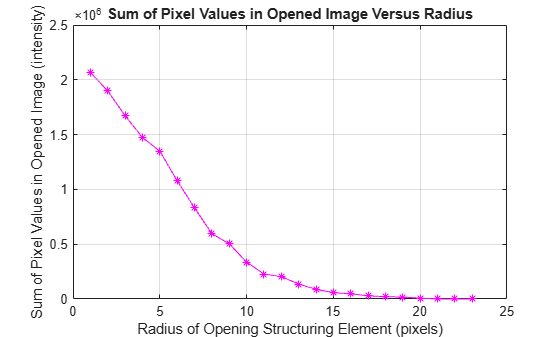### Extract Snowflakes Having a Particular Radius

Notice the minima and the radii where they occur in the graph. The minima tell you that snowflakes in the image have those radii. The more negative the minimum point, the higher the snowflakes' cumulative intensity at that radius. For example, the most negative minimum point occurs at the 5 pixel radius mark. You can extract the snowflakes having a 5 pixel radius with the following steps.

```open5 = imopen(claheI,strel('disk',5)); open6 = imopen(claheI,strel('disk',6)); rad5 = imsubtract(open5,open6); imshow(rad5,[])```